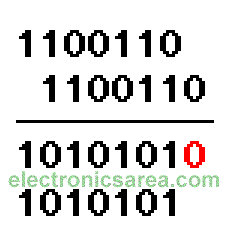# Binary to Gray Code Conversion and Gray code to Binary Conversion

We recommend reading the The Binary Number System before you start reading this tutorial.

## Binary to GRAY code Conversion

To convert Binary into Gray code, use the following method:

1 – We add the binary number to another like it. The second number need to be moved one digit to the right. See the chart.

2 – We do the binary addition digit by digit, and we discard the carry.

3 – We remove the last digit on the right side of the result on step 2 (we remove the zero which is in red color). The resulting code is the GRAY code.## GRAY code to Binary Conversion

To convert a GRAY code to a binary number, we use the following method:

1. The first digit of the Gray code will be the same in the binary number
2.  a) If the second digit of the Gray code is “0”, the second digit of the binary number is equal to the first digit.
b) If the second digit of the Gray code is “1”, the second digit of the binary number is the inverse of the first digit.
3. If the third digit of the Gray code is “0”, the third digit of the binary múmero equals the second digit of the binary number.
a) If the third digit of the Gray code is “1”, the third digit of the binary number is the inverse of the second binary number digit ….. and so continue until finished.
•
•
•
•
•
•
•
•
•
•
•
•
•
•
•
•
•
•